How Cheenta works to ensure student success?
Explore the Back-Story

# Test of Mathematics Solution Subjective 84 - Comparing EquationsThis is a Test of Mathematics Solution Subjective 84 (from ISI Entrance). The book, Test of Mathematics at 10+2 Level is Published by East West Press. This problem book is indispensable for the preparation of I.S.I. B.Stat and B.Math Entrance.

## Problem

Show that there is exactly one value of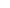that satisfies the equation: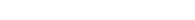## Solution:

We know that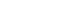for all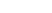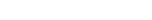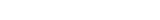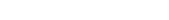Now consider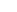and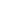. By AM-GM inequality we have,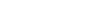So, only when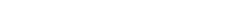.

That means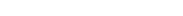. So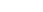being the only solution.

andalso satisfies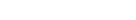Thus there is exactly one solution.

Hence Proved.This is a Test of Mathematics Solution Subjective 84 (from ISI Entrance). The book, Test of Mathematics at 10+2 Level is Published by East West Press. This problem book is indispensable for the preparation of I.S.I. B.Stat and B.Math Entrance.

## Problem

Show that there is exactly one value ofthat satisfies the equation:## Solution:

We know thatfor allNow considerand. By AM-GM inequality we have,So, only when.

That means. Sobeing the only solution.

andalso satisfiesThus there is exactly one solution.

Hence Proved.

### Knowledge Partner